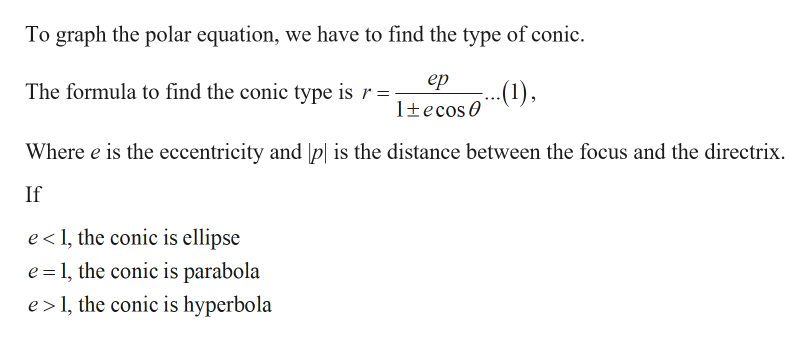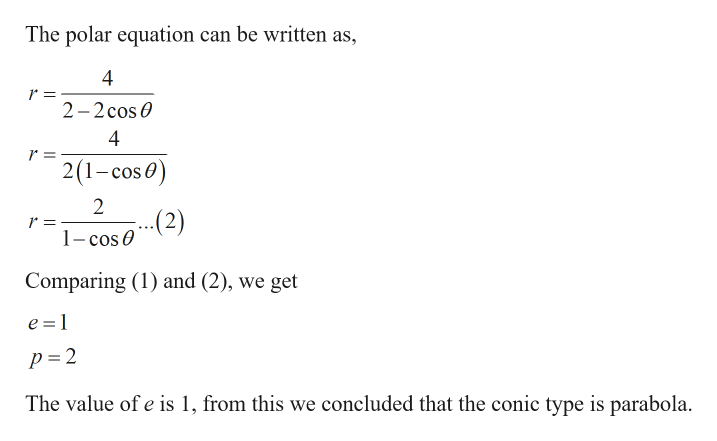# Graph the polar equationr=4/ 2-2 cos theta

Question
11 views

Graph the polar equation

r=4/ 2-2 cos theta

check_circle

Step 1

Given:

Step 2

Calculation:help_outlineImage TranscriptioncloseTo graph the polar equation, we have to find the type of conic ер ...) The formula to find the conic type is r 1tecos Where e is the eccentricity and p| is the distance between the focus and the directrix If e 1, the conic is ellipse e 1, the conic is parabola e 1, the conic is hyperbola fullscreen
Step 3help_outlineImage TranscriptioncloseThe polar equation can be written as 4 r = 2-2cos 4 r = 21-cos) 2 (2) 1-cos 0 Comparing (1) and (2), we get e =1 p =2 The value of e is 1, from this we concluded that the conic type is parabola fullscreen

### Want to see the full answer?

See Solution

#### Want to see this answer and more?

Solutions are written by subject experts who are available 24/7. Questions are typically answered within 1 hour.*

See Solution
*Response times may vary by subject and question.
Tagged in

### Calculus